## Sunday, September 11, 2016

### Interesting failure to find the ML transition rates in `fitMk` and `ace`

A phytools user reported a strange result from ancestral character reconstruction. I don't have a fix, per se, but it was interesting to diagnose and so I decided to (with his permission) share it here.

Firstly, let's take a look at our data. This is a real empirical dataset, but the names of the taxa have been anonymized to conceal any pre-publication results of this research:

``````library(phytools)
tree
``````
``````##
## Phylogenetic tree with 271 tips and 270 internal nodes.
##
## Tip labels:
##  t1, t2, t3, t4, t5, t6, ...
##
## Rooted; includes branch lengths.
``````
``````y
``````
``````##   t1   t2   t3   t4   t5   t6   t7   t8   t9  t10  t11  t12  t13  t14  t15
##    0    0    0    0    0    0    0    0    0    0    0    0    0    0    0
##  t16  t17  t18  t19  t20  t21  t22  t23  t24  t25  t26  t27  t28  t29  t30
##    0    1    1    0    0    0    0    0    0    0    0    0    1    0    0
##  t31  t32  t33  t34  t35  t36  t37  t38  t39  t40  t41  t42  t43  t44  t45
##    0    0    0    0    0    0    0    0    0    0    1    0    0    0    0
##  t46  t47  t48  t49  t50  t51  t52  t53  t54  t55  t56  t57  t58  t59  t60
##    0    0    0    0    0    0    0    0    0    0    0    0    0    0    0
##  t61  t62  t63  t64  t65  t66  t67  t68  t69  t70  t71  t72  t73  t74  t75
##    1    0    0    0    0    0    0    0    0    0    0    0    0    0    0
##  t76  t77  t78  t79  t80  t81  t82  t83  t84  t85  t86  t87  t88  t89  t90
##    0    1    0    0    0    0    0    0    0    0    1    0    1    0    0
##  t91  t92  t93  t94  t95  t96  t97  t98  t99 t100 t101 t102 t103 t104 t105
##    0    0    0    0    0    0    0    0    1    0    0    0    0    0    0
## t106 t107 t108 t109 t110 t111 t112 t113 t114 t115 t116 t117 t118 t119 t120
##    0    0    0    0    0    0    0    0    0    0    0    0    1    1    0
## t121 t122 t123 t124 t125 t126 t127 t128 t129 t130 t131 t132 t133 t134 t135
##    1    0    0    1    0    0    0    1    1    1    1    0    0    0    0
## t136 t137 t138 t139 t140 t141 t142 t143 t144 t145 t146 t147 t148 t149 t150
##    0    1    1    0    0    1    0    1    1    0    1    1    1    1    1
## t151 t152 t153 t154 t155 t156 t157 t158 t159 t160 t161 t162 t163 t164 t165
##    1    1    0    1    1    0    1    1    1    0    1    1    0    1    0
## t166 t167 t168 t169 t170 t171 t172 t173 t174 t175 t176 t177 t178 t179 t180
##    1    1    1    0    0    0    1    0    1    0    1    0    0    0    0
## t181 t182 t183 t184 t185 t186 t187 t188 t189 t190 t191 t192 t193 t194 t195
##    0    0    0    1    1    0    0    0    1    0    0    0    1    1    0
## t196 t197 t198 t199 t200 t201 t202 t203 t204 t205 t206 t207 t208 t209 t210
##    0    0    0    0    0    1    1    0    1    1    0    0    0    1    1
## t211 t212 t213 t214 t215 t216 t217 t218 t219 t220 t221 t222 t223 t224 t225
##    1    1    0    0    0    0    0    1    0    0    0    0    0    0    0
## t226 t227 t228 t229 t230 t231 t232 t233 t234 t235 t236 t237 t238 t239 t240
##    0    0    1    1    1    0    0    1    0    1    1    0    1    1    1
## t241 t242 t243 t244 t245 t246 t247 t248 t249 t250 t251 t252 t253 t254 t255
##    1    1    1    0    1    1    0    0    0    0    1    0    0    0    0
## t256 t257 t258 t259 t260 t261 t262 t263 t264 t265 t266 t267 t268 t269 t270
##    0    0    0    0    0    0    1    0    0    0    0    0    0    0    0
## t271
##    0
## Levels: 0 1
``````

We can plot these on the tree just as easily:

``````plotTree(tree,type="fan",lwd=1,ftype="off")
tiplabels(pie=to.matrix(y[tree\$tip.label],c(0,1)),
piecol=c("blue","red"),cex=0.3)
c(0,1)),x=-176,y=169,prompt=FALSE,shape="circle")
``````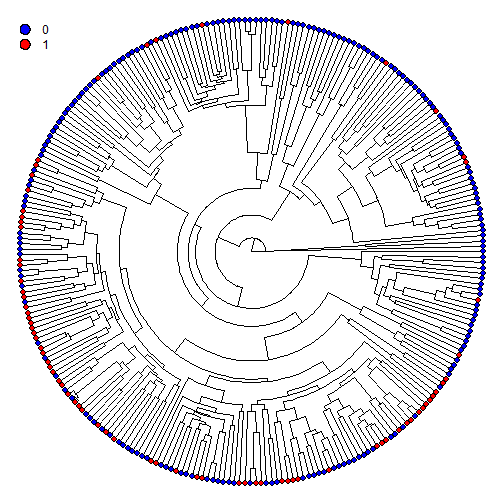and we see a character pattern that seems consistent with relatively conservative evolution of the trait.

However, when we estimate ancestral states using `ace` a very peculiar result emerges - basically all the internal nodes have marginal likelihoods of `0.5` of being in each of the two states:

``````plotTree(tree,type="fan",lwd=1,ftype="off")
tiplabels(pie=to.matrix(y[tree\$tip.label],c(0,1)),
piecol=c("blue","red"),cex=0.3)
c(0,1)),x=-176,y=169,prompt=FALSE,shape="circle")
fit.ace<-ace(y,tree,type="discrete")
nodelabels(pie=fit.ace\$lik.anc,piecol=c("blue",
"red"),cex=0.4)
``````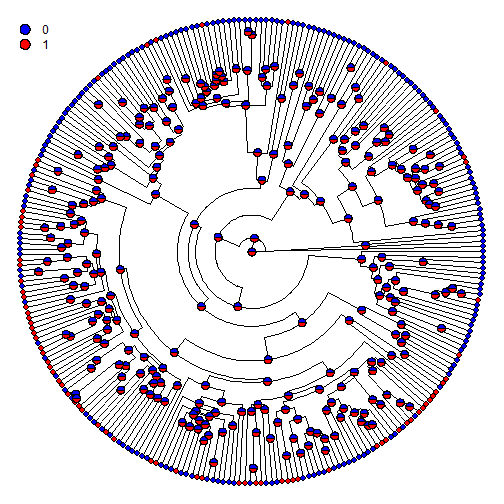We also find the same result using stochastic mapping:

``````mtrees<-make.simmap(tree,y,nsim=100)
``````
``````## make.simmap is sampling character histories conditioned on the transition matrix
##
## Q =
##           0         1
## 0 -1.100016  1.100016
## 1  1.100016 -1.100016
## (estimated using likelihood);
## and (mean) root node prior probabilities
## pi =
##   0   1
## 0.5 0.5
``````
``````## Done.
``````
``````plot(mtrees[],colors=setNames(c("blue","red"),c(0,1)),
ftype="off",type="fan")
``````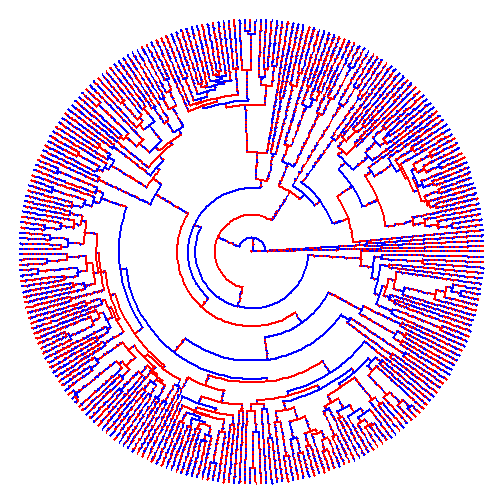It might be hard to tell, but there are (quite literally) thousands of changes between states mapped on this tree! When we use our stochastic maps to reconstruct posterior probabilities at nodes, the results converge on what we saw before:

``````obj<-summary(mtrees)
obj
``````
``````## 100 trees with a mapped discrete character with states:
##  0, 1
##
## trees have 14970.19 changes between states on average
##
## changes are of the following types:
##          0,1     1,0
## x->y 7453.55 7516.64
##
## mean total time spent in each state is:
##                 0            1    total
## raw  6834.9706198 6772.2170663 13607.19
## prop    0.5023059    0.4976941     1.00
``````
``````plot(obj,type="fan",ftype="off",lwd=1,cex=c(0.4,0.3),
colors=setNames(c("blue","red"),c(0,1)))
``````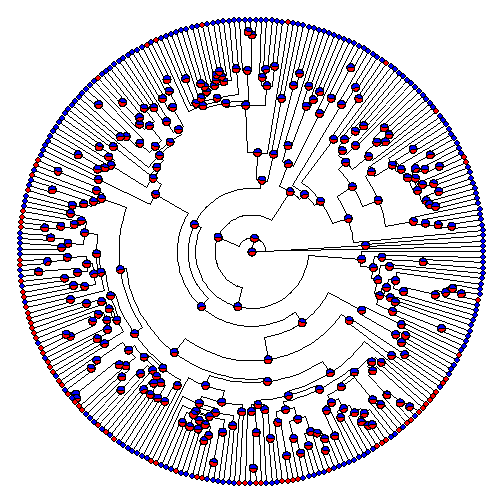So, what's going on?

Well, it turns out we can show that the fitted rate of transition between states obtained by `ace` (or by `fitMk`, which is used by `make.simmap` internally) is too high.

``````fit.ace
``````
``````##
##     Ancestral Character Estimation
##
## Call: ace(x = y, phy = tree, type = "discrete")
##
##     Log-likelihood: -187.1497
##
## Rate index matrix:
##   0 1
## 0 . 1
## 1 1 .
##
## Parameter estimates:
##  rate index estimate std-err
##           1      1.1 25.9547
##
## Scaled likelihoods at the root (type '...\$lik.anc' to get them for all nodes):
##   0   1
## 0.5 0.5
``````
``````fit.phytools<-fitMk(tree,y)
fit.phytools
``````
``````## Object of class "fitMk".
##
## Fitted (or set) value of Q:
##           0         1
## 0 -1.100016  1.100016
## 1  1.100016 -1.100016
##
## Fitted (or set) value of pi:
##   0   1
## 0.5 0.5
##
## Log-likelihood: -187.842892
``````

We'll do this by visualizing the likelihood surface from a number just above zero, to a value about 10% larger than the estimated rate, as follows:

``````q<-seq(0.001,1.2,by=0.001)
logL<-sapply(q,function(q,tree,x)
logLik(fitMk(tree,x,fixedQ=matrix(c(-q,q,q,-q),2,2))),
tree=tree,x=y)
plot(q,logL,type="l",xlab="q(0,1) & q(1,0)",ylab="log(L)")
abline(v=fit.phytools\$rates,lty="dashed")
``````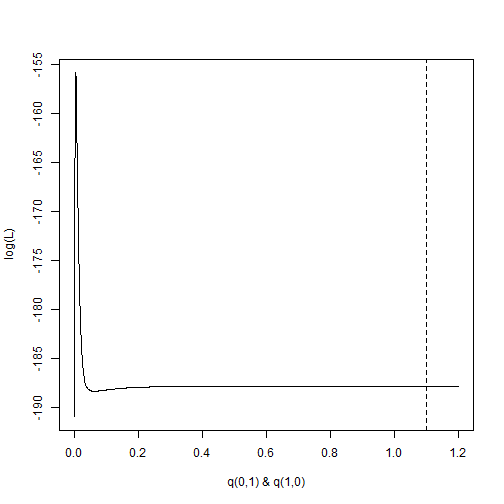It's pretty plain to see that the likelihood is not maximized at q0,1 = 1.1, but rather at a much smaller value:

``````ii<-which(logL==max(logL))
q[ii]
``````
``````##  0.005
``````

Furthermore, the reason optimization goes awry is because, after the initial peak, the likelihood monotically increases for increasing values of the transition rate, q.

Now, if we force the transition rate to this better value, we obtain much more reasonable results. For instance:

``````q<-q[ii]
Q=matrix(c(-q,q,q,-q),2,2)
rownames(Q)<-colnames(Q)<-c(0,1)
mtrees<-make.simmap(tree,y,nsim=100,Q=Q)
``````
``````## make.simmap is sampling character histories conditioned on the transition matrix
##
## Q =
##        0      1
## 0 -0.005  0.005
## 1  0.005 -0.005
## (specified by the user);
## and (mean) root node prior probabilities
## pi =
##   0   1
## 0.5 0.5
``````
``````## Done.
``````
``````plot(mtrees[],colors=setNames(c("blue","red"),c(0,1)),
ftype="off",type="fan")
``````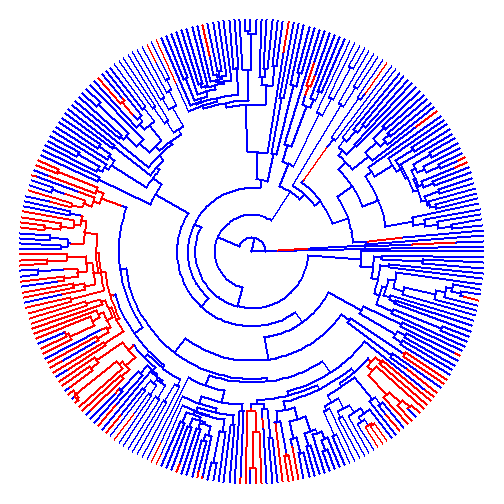or:

``````obj<-summary(mtrees)
obj
``````
``````## 100 trees with a mapped discrete character with states:
##  0, 1
##
## trees have 68.06 changes between states on average
##
## changes are of the following types:
##        0,1   1,0
## x->y 46.35 21.71
##
## mean total time spent in each state is:
##                 0            1    total
## raw  1.091646e+04 2690.7285272 13607.19
## prop 8.022568e-01    0.1977432     1.00
``````
``````plot(obj,type="fan",ftype="off",lwd=1,cex=c(0.4,0.3),
colors=setNames(c("blue","red"),c(0,1)))
``````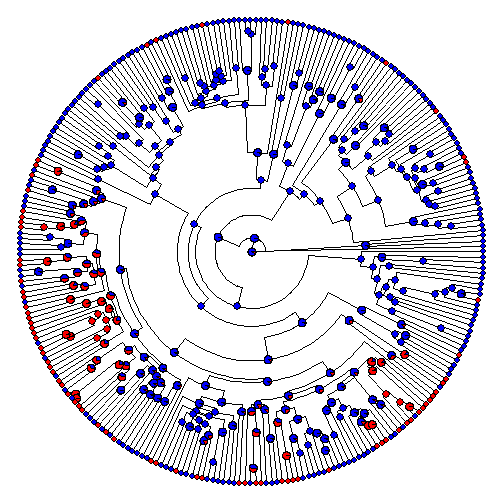In this case, `fitDiscrete` in the geiger package does converge on the right solution:

``````library(geiger)
fitDiscrete(tree,y)
``````
``````## GEIGER-fitted comparative model of discrete data
##  fitted Q matrix:
##                  0            1
##     0 -0.004976043  0.004976043
##     1  0.004976043 -0.004976043
##
##  model summary:
##  log-likelihood = -155.337575
##  AIC = 312.675150
##  AICc = 312.690020
##  free parameters = 1
##
## Convergence diagnostics:
##  optimization iterations = 100
##  failed iterations = 0
##  frequency of best fit = 1.00
##
##  object summary:
##  'lik' -- likelihood function
##  'bnd' -- bounds for likelihood search
##  'res' -- optimization iteration summary
##  'opt' -- maximum likelihood parameter estimates
``````

Finally, we also get the right ancestral states if we just rescale the tree. This is because then the estimated transition rates are much higher, but the likelihood & posterior probabilities at nodes remain unchanged. For instance:

``````RESCALE<-function(tree,h=1){
tree\$edge.length<-tree\$edge.length/max(nodeHeights(tree))*h
tree
}
fit.rescale<-ace(y,RESCALE(tree),type="discrete")
fit.rescale
``````
``````##
##     Ancestral Character Estimation
##
## Call: ace(x = y, phy = RESCALE(tree), type = "discrete")
##
##     Log-likelihood: -155.2032
##
## Rate index matrix:
##   0 1
## 0 . 1
## 1 1 .
##
## Parameter estimates:
##  rate index estimate std-err
##           1   0.8832  0.1055
##
## Scaled likelihoods at the root (type '...\$lik.anc' to get them for all nodes):
##          0          1
## 0.93206197 0.06793803
``````
``````plotTree(tree,type="fan",lwd=1,ftype="off")
tiplabels(pie=to.matrix(y[tree\$tip.label],c(0,1)),
piecol=c("blue","red"),cex=0.3)
c(0,1)),x=-176,y=169,prompt=FALSE,shape="circle")
nodelabels(pie=fit.rescale\$lik.anc,piecol=c("blue",
"red"),cex=0.4)
``````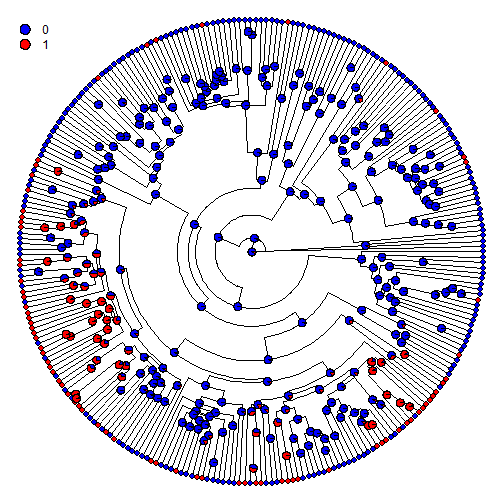Now to figure out what can be done to ensure that `fitMk` finds the right solution in the first place!

1.1.